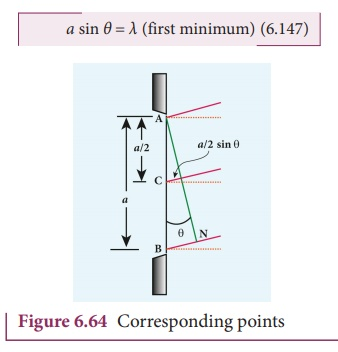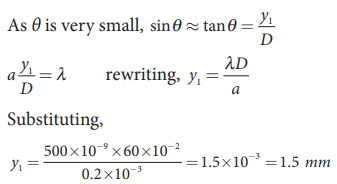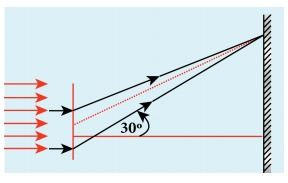Home | | Physics 12th Std | Diffraction at single slit

# Diffraction at single slit

The diffracted beam falls on a screen kept at a distance. The center of the slit is C. A straight line through C perpendicular to the plane of slit meets the center of the screen at O.

Diffraction at single slit

Let a parallel beam of light fall normally on a single slit AB of width a as shown in Figure 6.63. The diffracted beam falls on a screen kept at a distance. The center of the slit is C. A straight line through perpendicular to the plane of slit meets the center of the screen at O. We would like to find the intensity at any point on the screen. The lines joining to the different points on the slit can be treated as parallel lines, making an angle ╬Ė with the normal CO.

All the waves start parallel to each other from different points of the slit and interfere at point and other points to give the resultant intensities. The point is in the geometrically shadowed region, up to which the central maximum is spread due to diffraction as shown Figure 6.63. We need to give the condition for the point to be of various minima.The basic idea is to divide the slit into much smaller even number of parts. Then, add their contributions at with the proper path difference to show that destructive interference takes place at that point to make it minimum. To explain maximum, the slit is divided into odd number of parts.

### Condition for P to be first minimum

Let us divide the slit AB into two half ŌĆÖs AC and CB. Now the width of AC is (a/2). We have different points on the slit which are separated by the same width (here a/2) called corresponding points as shown in Figure 6.64.

The path difference of light waves from different corresponding points meeting at point P and interfere destructively to make it first minimum. The path difference ╬┤ between waves from these corresponding points is, ╬┤ = a/2 sin╬Ė

The condition for P to be first minimum, a/2 sin╬Ė = ╬╗/2Condition for P to be second minimum

Let us divide the slit AB into four equal parts. Now, the width of each part is a/4. We have several corresponding points on the slit which are separated by the same width a/4. The path difference ╬┤ between waves from these corresponding points is, ╬┤ = a/4 sin╬Ė.

The condition for to be second minimum, a/4 sin╬Ė = ╬╗/2

sin ╬Ė = 2╬╗ (second minimum)                  (6.148)### Condition for P to be third order minimum

The same way the slit is divided in to six equal parts to explain the condition for to be third minimum is, a/6 sin╬Ė = ╬╗/2sin ╬Ė = 3╬╗ (third minimum) (6.149)

### Condition for P to be nthorder minimum

Dividing the slit into 2number of (even number of) equal parts makes the light produced by one of the corresponding points to be cancelled by its counterpart. Thus, the condition for nth order minimum is a/2n sin╬Ė = ╬╗/2

sin ╬Ė n╬╗ (nth minimum)       (6.150)### Condition for maxima

For points of maxima, the slit is to be divided in to odd number of equal parts so that one part remains un-cancelled making the point appear bright.

The condition for first maximum is,In the same way, condition for nth maximum is,where, = 0, 1, 2, 3, . . . , is the order of diffraction maximum.

The central maximum is called 0th order maximum. The points of the maximum intensity lie nearly midway between the successive minima.

Here, sin╬Ė gives the angular spread of the diffraction. The position of the minimum or maximum in terms of y may be expressed by replacing sin╬Ė approximated by tan╬Ė, as ╬Ė is small, sin╬Ė = tan╬Ė y/D

Where, y is the position of the minimum from the center of the screen and D is the distance between single slit and the screen.

EXAMPLE 6.31

Light of wavelength 500 nm passes through a slit of 0.2 mm wide. The diffraction pattern is formed on a screen 60 cm away. Determine the,

(i) angular spread of central maximum

(ii) the distance between the central maximum and the second minimum.

Solution

╬╗ = 500 nm = 500├Ś10-9 m; = 0.2 mm = 0.2├Ś10-3 m; = 60 cm = 60├Ś10-2 m

(i) Equation for diffraction minimum is, sin ╬Ė n╬╗

The central maximum is spread up to the first minimum. Hence, = 1(ii) To find the value of y1 for central maximum, which is spread up to first minimum with (= 1) is, sin ╬Ė ╬╗To find the value of y2 for second minimum with (n = 2) is, a sin ╬Ė = 2╬╗The distance between the central maximum and second minimum is, y2 ŌĆō y1

y2 ŌĆō y1 = 3 mm ŌĆō 1.5 mm = 1.5 mmNote: The above calculation shows that in the diffraction pattern caused by single slit, the width of each maximum is equal with central maximum as the double that of others. But the bright and dark fringes are not of equal width.

EXAMPLE 6.32

A monochromatic light of wavelength 5000 ├ģ passes through a single slit producing diffraction pattern for the central maximum as shown in the figure. Determine the width of the slit.Solution

╬╗ = 5000 ├ģ = 5000├Ś10-10 m; sin 30┬║ = 0.5; = 1; =?

Equation for diffraction minimum is, asin ╬Ė n╬╗

The central maximum is spread up to the first minimum. Hence, = 1

Rewriting, = ╬╗ / Sin╬Ė

Substituting, = 5000├Ś10ŌłÆ10 / 0.5

= 1├Ś10ŌłÆ6 = 0.001├Ś10ŌłÆ3= 0.001mm

## Discussion on first minimum

Let us consider the condition for first minimum with (= 1). sin ╬Ė ╬╗

The first minimum has an angular spread of, sin╬Ė = ╬╗/a

Now, we have special cases to discuss on the above condition.

(i) When ╬╗, the diffraction is not possible, because sin╬Ė can never be greater than 1.

(ii) When a Ōēź ╬╗, the diffraction is possible.

ŌŚŚ For a = ╬╗, sin╬Ė = 1 i.e, ╬Ė = 90┬║. That means the first minimum is at 90┬║. Hence, the central maximum spreads fully in to the geometrically shadowed region leading to bending of the diffracted light to 90┬║.

ŌŚŚ For >> ╬╗, sin╬Ė << 1 i.e, the first minimum will fall within the width of the slit itself. The diffraction will not be noticed at all.

(iii) When ╬╗ and also comparable, say a = 2╬╗, sin╬Ė = ╬╗/a = ╬╗/2╬╗ = 1/2; then ╬Ė = 30┬║. These are practical cases where diffraction could be observed effectively.

Study Material, Lecturing Notes, Assignment, Reference, Wiki description explanation, brief detail
12th Physics : UNIT 7 : Wave Optics : Diffraction at single slit |